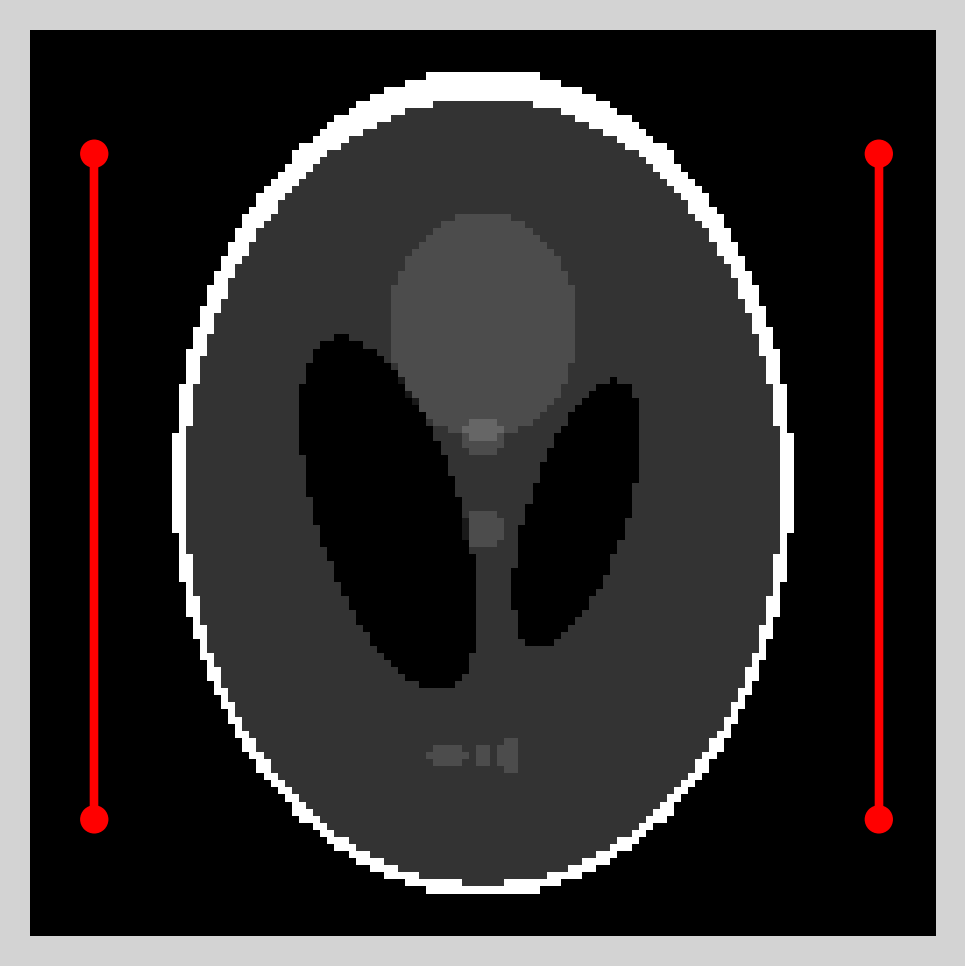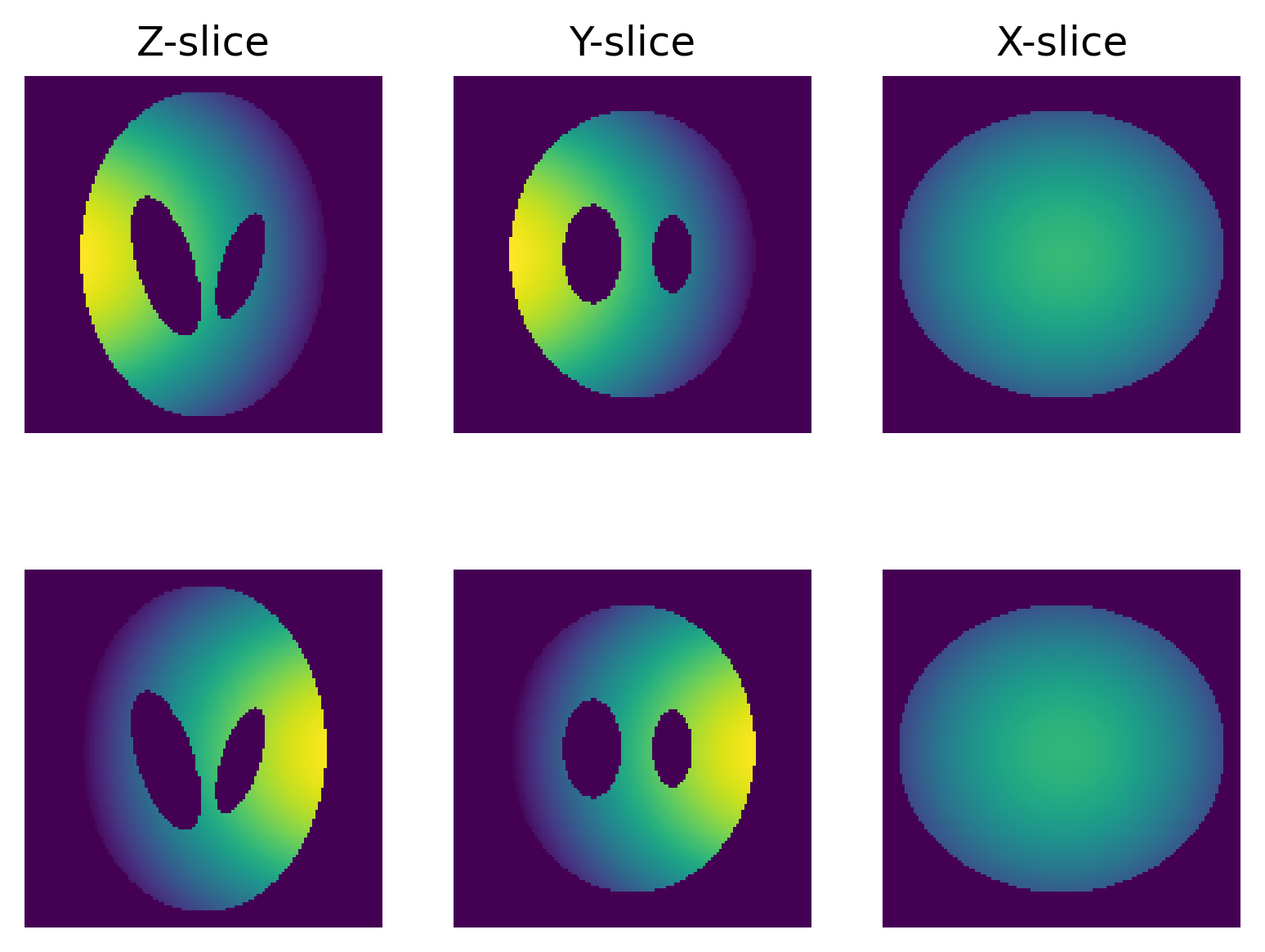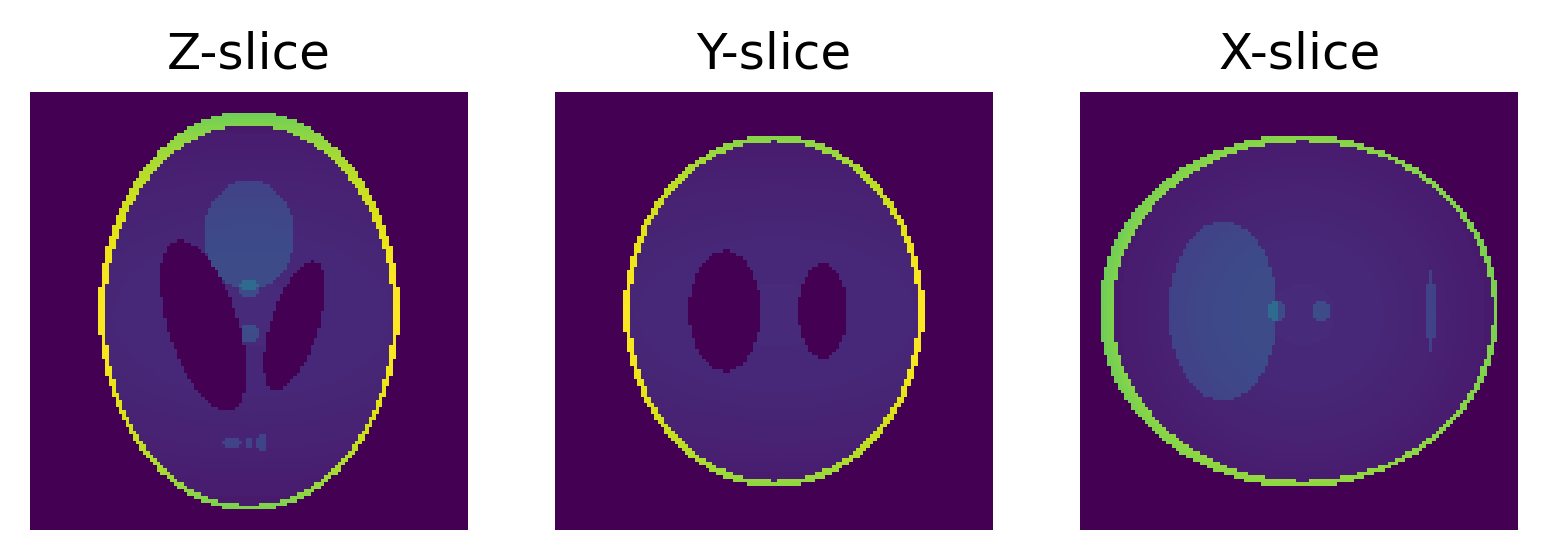Utility functions to produce and visualize simulated bias fields generated according to Kern et. al's sinusoidal sensitivity model.

# biasgen

biasgen is a Python package which simulates magnetic resonance (MR) non-uniformities. This package allows users to generate custom bias fields by combining radiofrequency (RF) coil spatial information with frequency sampling information.

Bias fields are constructed using a sum-of-squares approach with coil sensitivity maps which follow the sinusoidal model of Kern et. al. Direct computation of sinusoidal sensitivities are done in closed form using the segment source solution of Vinas and Sudhyadhom.

# Installation

Package biasgen can be installed using pip:

pip install biasgen


A CUDA accelerated version of biasgen is also available:

pip install biasgen[gpu]


# Usage

To start biasgen requires the user to define a set of coil positions and sampling frequencies. Coil positioning can be initialized through a sequence of CoilSegment objects or by using the predefined coil constructor routine cage_constructor. An example of how to use cage_constructor is provided below:

import biasgen

# Shepp-Logan phantom example
ph = biasgen.phantom3d(n=128)

# returns a sequence of CoilSegment objects
coils = biasgen.cage_constructor(n_coils=2, center=(64,64,64), coil_height=128,
length_to_space_ratio=0.35, ellip_axes=(90,65))

biasgen.view_coil_positioning(ph, coils)Function view_coil_positioning provides a top-down view of CoilSegment sequences which can be useful when arranging coil cages. Next step is to define a sampling grid for the sinusoidal sensitivity model:

sens_settings = biasgen.SensitivitySettings(grid_lengths=(5,5,5), grid_spacings=(1,1,1))


Inputting the sampling information and coil sequence into compute_sensitivity will return the sensitivity maps of each coils:

# biasgen.use_gpu(True) # Uncomment if GPU is available

# first axis will index each coil contribution
sens = biasgen.compute_sensitivity(coils, sens_settings, ph.shape, batch_sz=1, scale_fctr=0.5)


Arguments "batch_sz" and "scale_fctr" can be helpful for memory-limited devices. "batch_sz" determines how many segments are loaded into memory while "scale_fctr" provides a temporary spatial downsampling for computation. The simulated sensitivity maps can be viewed using the function view_center_axes:

# mask for view_center_axes

# visualization requires that a mask is providedFinally we construct the measured bias field through a sum-of-squares procedure:

bias = biasgen.bias_sum_of_squares(sens)

# scalar product application of bias to Shepp-Logan phantomMore detailed examples of sensitivity simualtions and visualizaitons can be found in the examples/bias.ipynb notebook.

# References

1. Guerquin-Kern M, Lejeune L, Pruessmann KP, Unser M. Realistic Analytical Phantoms for Parallel Magnetic Resonance Imaging. IEEE Transactions on Medical Imaging. 2012;31(3):626-636.

2. Vinas L and Sudhyadhom A. Sinusoidal Sensitivity Calculation for Line Segment Geometries. arXiv.org:2208.03059 [physics.med-ph], Aug. 2022.

## Project details

This version0.1.30.1.20.1.10.1.0

Uploaded source
Uploaded py3